# Lateral surface area

The ratio of the area of the base of the rotary cone to its lateral surface area is 3: 5. Calculate the surface and volume of the cone, if its height v = 4 cm.

Correct result:

S =  75.398 cm2
V =  37.699 cm3

#### Solution:

$v=4 \ \text{cm} \ \\ S_{1}:S_{2}=3:5 \ \\ \ \\ S_{1}=\pi r^2 \ \\ S_{2}=\pi r s \ \\ \ \\ 3/5=\dfrac{ \pi r^2 }{ \pi r s } \ \\ 3/5=r/s \ \\ \ \\ s^2=r^2 + v^2 \ \\ s^2=(3/5s)^2 + v^2 \ \\ \ \\ s=v / \sqrt{ 1 - (3/5)^{ 2 } }=4 / \sqrt{ 1 - (3/5)^{ 2 } }=5 \ \text{cm} \ \\ \ \\ r=3/5 \cdot \ s=3/5 \cdot \ 5=3 \ \text{cm} \ \\ \ \\ S_{1}=\pi \cdot \ r ^2=3.1416 \cdot \ 3 ^2 \doteq 28.2743 \ \text{cm}^2 \ \\ S_{2}=\pi \cdot \ r \cdot \ s=3.1416 \cdot \ 3 \cdot \ 5 \doteq 47.1239 \ \text{cm}^2 \ \\ \ \\ k=S_{1}/S_{2}=28.2743/47.1239=\dfrac{ 3 }{ 5 }=0.6 \ \\ \ \\ S=S_{1}+S_{2}=28.2743+47.1239=75.398 \ \text{cm}^2$
$V=\dfrac{ 1 }{ 3 } \cdot \ S_{1} \cdot \ v=\dfrac{ 1 }{ 3 } \cdot \ 28.2743 \cdot \ 4=37.699 \ \text{cm}^3$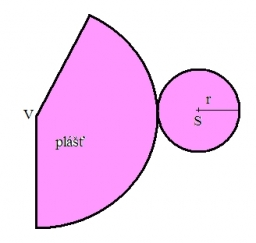Our examples were largely sent or created by pupils and students themselves. Therefore, we would be pleased if you could send us any errors you found, spelling mistakes, or rephasing the example. Thank you!

Please write to us with your comment on the math problem or ask something. Thank you for helping each other - students, teachers, parents, and problem authors.Tips to related online calculators
Check out our ratio calculator.
Tip: Our volume units converter will help you with the conversion of volume units.
Pythagorean theorem is the base for the right triangle calculator.

#### You need to know the following knowledge to solve this word math problem:

We encourage you to watch this tutorial video on this math problem:

## Next similar math problems:In a regular quadrilateral pyramid, the height is 6.5 cm and the angle between the base and the side wall is 42°. Calculate the surface area and volume of the body. Round calculations to 1 decimal place.A regular quadrilateral pyramid has a volume of 24 dm3 and a base edge a = 4 dm. Calculate: a/height of the pyramid b/sidewall height c/surface of the pyramid
• Triangular prismThe triangular prism has a base in the shape of a right triangle, the legs of which is 9 cm and 40 cm long. The height of the prism is 20 cm. What is its volume cm3? And the surface cm2?
• The regularThe regular triangular prism has a base in the shape of an isosceles triangle with a base of 86 mm and 6.4 cm arms, the height of the prism is 24 cm. Calculate its volume.What is the volume of a quadrilateral oblique prism with base edges of length a = 1m, b = 1.1m, c = 1.2m, d = 0.7m, if a side edge of length h = 3.9m has a deviation from the base of 20° 35 ´ and the edges a, b form an angle of 50.5°.
• Volume ratio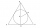Calculate the volume ratio of balls circumscribed (diameter r) and inscribed (diameter ϱ) into an equilateral rotating cone.
• Mixing 5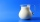Carlos mixed 4/15 of chocolate syrup with 1/2 of milk. Determine the reasonable estimate of the total amount of liquid
• Truncated cone 6Calculate the volume of the truncated cone whose bases consist of an inscribed circle and a circle circumscribed to the opposite sides of the cube with the edge length a=1.
• Long drive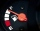Calculate how much it costs to drive 150,000 km with an average consumption of 7 liters per 100 km and a fuel price of 1.2 € / l.
• Company carMs. Vaňková has a company car equipped with a multifunction indicator, on which she can monitor the average petrol consumption during individual rides. During the first ride, mostly in city traffic, it traveled 20.5 km, with an average consumption of 7.8L
• Gas, lpg and diesel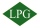Calculate how much it costs to drive 35000 km by car: a) a car with a consumption of 8l/100 km of LPG for 60 cents b) 7l/100 km of petrol for 1.33 euros c) 6 l/100 km of diesel for 1.2 euros. Calculate what is cheaper.
• Solutions, mixturesWe have 2 liters of 20% solution available. How much 70% solution do we need to add to it to get a 30% solution?
• Copper wireWhat is the mass of 500 m of copper wire with a diameter of 1 mm, if ρ = 8.9 g/cm ^ 3?
• Converting units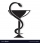Health care professionals frequently convert from one system of measurement to another. Whether you are converting a metric measurement into the household system for a patient or converting within the metric system to enter data in a specific format, the
• WeightThe kilogram weight has a volume of 116 ml. From which material is it probably made? Use substance density tables, e. G. mathematical-physical-chemical tables.
• Gas sphere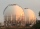The gas tank has the shape of a sphere with a diameter of 14 m. How many m3 of gas will fit in it?
• Clay balls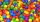How many clay balls with a radius of 1 cm can be made from a ball of clay with a radius of 8 cm?